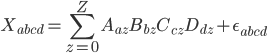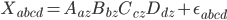2 views

I am trying to decompose a 3D matrix using python library scikit-tensor. I managed to decompose my Tensor (with dimensions 100x50x5) into three matrices. My question is how can I compose the initial matrix again using the decomposed matrix produced with Tensor factorization? I want to check if the decomposition has any meaning. My code is the following:

import logging

from sktensor import dtensor, cp_als

import numpy as np

//Set logging to DEBUG to see CP-ALS information

logging.basicConfig(level=logging.DEBUG)

T = np.ones((400, 50))

T = dtensor(T)

P, fit, itr, exectimes = cp_als(T, 10, init='random')

// how can I re-compose the Matrix T? TA = np.dot(P.U, P.U.T)

I am using the canonical decomposition as provided from the scikit-tensor library function cp_als. Also what is the expected dimensionality of the decomposed matrices?

by (33.1k points)

You can simply perform a cross product of matrics.can be expressed using Einstein notation asCode implementation of this problem using NumPy:

numpy.einsum('az,bz,cz,dz -> abcd', A, B, C, D)

so in your case, you would use

numpy.einsum('az,bz->ab', P.U, P.U)

numpy.einsum('az,bz,cz->abc', P.U, P.U, P.U)

sktensor.ktensor.ktensor also have a method totensor() that does exactly this:

np.allclose(np.einsum('az,bz->ab', P.U, P.U), P.totensor())

>>> True

If you want to be build successful data science career then enroll